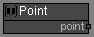# Point node

Left Toolbar >Math Nodes >Point

The Point node allows you to set a point x and y values. Default (0,0)

## Procedure

### To define a point in the node graph:

1. On the left toolbar choose Math Nodes> Point2. Drag the Point icon into the Node Graph.3. Connect the Output: point.

## Inputs

X

X position. A NUMBER type of value.

Y

Y position. A NUMBER type of value.

## Outputs

point

Point Value (x,y).

## Attributes PanelX

X value. This attribute is not exposed as input by default. Right-click the Node to expose this attribute as input.

Y

Y Value. This attribute is not exposed as input by default. Right-click the Node to expose this attribute as input.

Math Nodes

Main Page